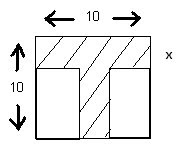## Thursday, September 11, 2008

Today Mrs.Ingram gave us work to do once we got into class and here are the questions if you didn't happen to get them EVEN though everyone got a text book, but here are the questions:

Exercise 3: Division by Binomials -1 ( 13-18 )

13)Simplify:

a) (x²)³ + (x³)²
b) (x+x+x)²

14)Simplify:

a)3-2[x-(4)]

15)A carpenter takes a piece of plywood measuring 10 units by 10 units and cuts out a letter T as shown. If the T is of a uniform width, x, write an algebraic expression for its area.16)ABCD is divided into 4 rectangles as shown. The areas of three rectangles are given. What is the area of the fourth rectangle?17)Simplify the expression:
a)(x+2)² - x²

18)Multiply:
a) 2x(x+1((x-3)

Exercise 8: Division by Binomials -2 (1-4)

1. Divide: 3x³+3x+2 by (x+3)

2. Divide: (4x³-1+8x) ÷ (4+4x)

3. Divide: (3x³+1+3x) by (6+3x)

4. Divide: (2x³+x+6) ÷ (x+1)